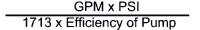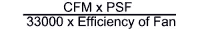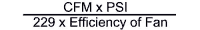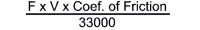INDUSTRIAL PROCESS INSTRUMENTS AND CONTROLS

## QUICK ESTIMATE FORMULAS

HORSEPOWER FORMULAS
For Rotating Objects

HP =Where T = Torque (lbft)
N = Speed (rpm)

Objects in Linear Motion HP =Where F = Force (lbs)
V = Velocity (ft/min)

For Pumps HP =HP =Where

GPM = Gallons per Minute
Head = Height of Water (ft)
Efficiency of Pump = %/100
PSI = Pounds per Inch

Specific Gravity of Water = 1.0
1 CuFt per Sec. = 448 GPM
1 PSI = A Head of 2.309 ft (water weight)
62.36 lbs per CuFt at 62°F

For Fans and Blowers HP =HP =HP =Where CFM = Cubic Feet per Minute
PSF = Pounds per Square Foot
PIW = Inches of Water Gauge
PSI = Pounds per Square Inch
Efficiency of Fan = %/100

For Conveyors HP (verticle)HP (horizontal)Where

F = Force (lbs)
V = Velocity (ft/min)

Coef. of Friction
• Ball or Roller Slide = 0.02
• Dovetail Slide = 0.20
• Hydrostatic Ways = 0.01
• Rectangle Ways with Gib = 0.01 to 0.25

TORQUE FORMULAS
T = HP x 5252

N

Where T = Torque (LbFt)
HP = Horsepower
N = Speed (rpm)

T = F x R
Where T = Torque (LbFt)
F = Force (Lbs)
R = Radius (Ft)

Ta (accelerating) WK2 x Change in RPM

308 x t (Sec)

Where

Ta = Torque (LbFt)
WK2 = Inertia at Motor Shaft (LbFt)2
t = Time to Accelerate (Sec)

Note:
To change LbFt2 to InLbSec2, Divide by 2.68
To change InLbSec2 to LbFt2, Mult. by 2.68

AC MOTOR FORMULAS
Sync Speed = Freq x 120
Number of Poles
Where Sync Speed = Synchronous Speed (Rpm)
Freq = Frequency (Hz)

% Slip = (Sync Speed - FL Speed) x 100

Sync Speed

Where FL Speed = Full Load Speed (Rpm)
Sync Speed = Synchronous Speed (Rpm)

Reflected WK2 =

(Reduction Ratio)2

 Go Shopping Search Products Basket Contents Track Order Company Information Terms & Conditions Your Privacy Manufacturers Contact Us! Technical Library Chemical Compatibility Cleanroom Standards Light Color Codes Conversion Charts Electrical Safety Electrical Symbols FCC Rules Heater Selection Humidity Motors Gears, Drives Ohm's Law Particle Contamination Power Equations Pressure Help Quick Formulas Research Links Temperature Conv t/c  Reference RTD Reference Time Standards 3-Phase Equations LonWorks Carbon Dioxide

SEARCH  INVENTORY